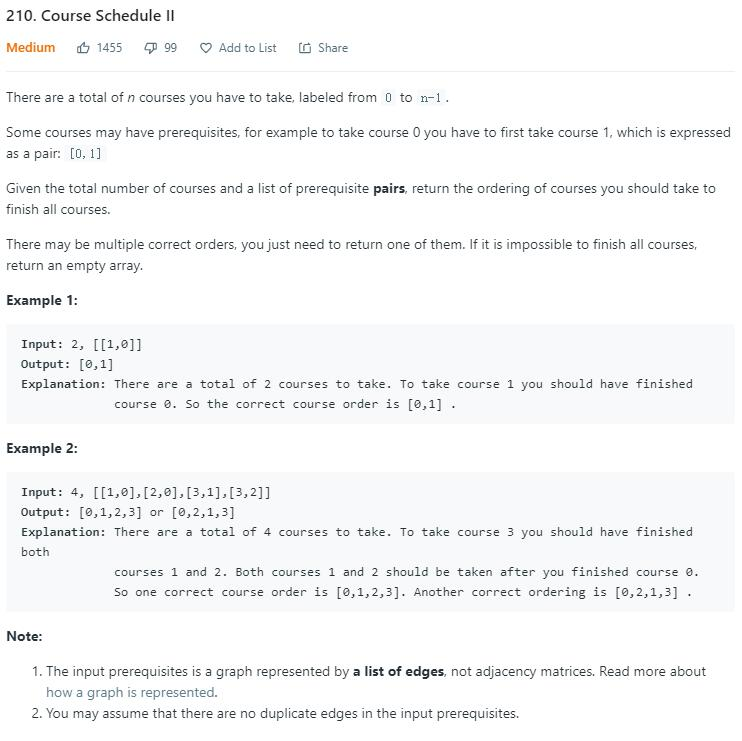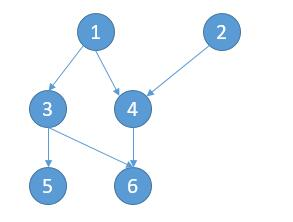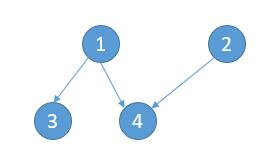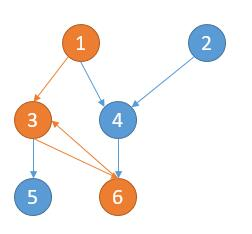# 题目描述（中等难度）207 题 Course Schedule 的延伸，给定 n 组先修课的关系，[m,n] 代表在上 m 这门课之前必须先上 n 这门课。输出一个上课序列。

# 思路分析

207 题 考虑是否存在一个序列上完所有课，这里的话，换汤不换药，完全可以按照 207 题 的解法改出来，大家可以先去看一下。主要是两种思路，BFSDFS ，题目就是在考拓扑排序。

# 解法一public int[] findOrder(int numCourses, int[][] prerequisites) {
// 保存每个节点的先修课个数，也就是出度
HashMap<Integer, Integer> outNum = new HashMap<>();
// 保存以 key 为先修课的列表，也就是入度的节点
HashMap<Integer, ArrayList<Integer>> inNodes = new HashMap<>();
// 保存所有节点
HashSet<Integer> set = new HashSet<>();
int rows = prerequisites.length;
for (int i = 0; i < rows; i++) {
int key = prerequisites[i];
int value = prerequisites[i];
if (!outNum.containsKey(key)) {
outNum.put(key, 0);
}
if (!outNum.containsKey(value)) {
outNum.put(value, 0);
}
// 当前节点先修课个数加一
int num = outNum.get(key);
outNum.put(key, num + 1);

if (!inNodes.containsKey(value)) {
inNodes.put(value, new ArrayList<>());
}
// 更新以 value 为先修课的列表
ArrayList<Integer> list = inNodes.get(value);
}

// 将当前先修课个数为 0 的课加入到队列中
for (int k : set) {
if (outNum.get(k) == 0) {
queue.offer(k);
}
}
int[] res = new int[numCourses];
int count = 0;
while (!queue.isEmpty()) {
// 队列拿出来的课代表要删除的节点
// 要删除的节点的 list 中所有课的先修课个数减一
int v = queue.poll();
//**************主要修改的地方********************//
res[count++] = v;
//**********************************************//
ArrayList<Integer> list = inNodes.getOrDefault(v, new ArrayList<>());

for (int k : list) {
int num = outNum.get(k);
// 当前课的先修课要变成 0, 加入队列
if (num == 1) {
queue.offer(k);
}
// 当前课的先修课个数减一
outNum.put(k, num - 1);
}
}
for (int k : set) {
if (outNum.get(k) != 0) {
//有课没有完成，返回空数组
return new int;
}
}
//**************主要修改的地方********************//
HashSet<Integer> resSet = new HashSet<>();
for (int i = 0; i < count; i++) {
}
//有些课是独立存在的，这些课可以随时上，添加进来
for (int i = 0; i < numCourses; i++) {
if (!resSet.contains(i)) {
res[count++] = i;
}
}
//**********************************************//
return res;
}


# 解法二1 -> 3 -> 51 -> 3 -> 61 -> 4 -> 6

2 -> 4 -> 6

3 -> 53 -> 6

4 -> 6

5

6public int[] findOrder(int numCourses, int[][] prerequisites) {
HashMap<Integer, ArrayList<Integer>> outNodes = new HashMap<>();
HashSet<Integer> set = new HashSet<>();
int rows = prerequisites.length;
for (int i = 0; i < rows; i++) {
int key = prerequisites[i];
int value = prerequisites[i];
if (!outNodes.containsKey(key)) {
outNodes.put(key, new ArrayList<>());
}
// 存储当前节点的所有先修课程
ArrayList<Integer> list = outNodes.get(key);
}

int[] res = new int[numCourses];
HashSet<Integer> resSet = new HashSet<>(); //防止重复的节点加入
HashSet<Integer> visitedFinish = new HashSet<>();
// 判断每一门课
for (int k : set) {
if (!dfs(k, outNodes, new HashSet<>(), visitedFinish, res, resSet)) {
return new int;
}
}
//和之前一样，把独立的课加入
for (int i = 0; i < numCourses; i++) {
if (!resSet.contains(i)) {
res[count++] = i;
}
}
return res;
}

int count = 0;

private boolean dfs(int start, HashMap<Integer, ArrayList<Integer>> outNodes, HashSet<Integer> visited,
HashSet<Integer> visitedFinish, int[] res, HashSet<Integer> resSet) {
// 已经处理过
if (visitedFinish.contains(start)) {
return true;
}
//**************主要修改的地方********************//
// 到了叶子节点
if (!outNodes.containsKey(start)) {
if (!resSet.contains(start)) {
res[count++] = start;
}
return true;
}
//**********************************************//
// 出现了环
if (visited.contains(start)) {
return false;
}
// 将当前节点加入路径
ArrayList<Integer> list = outNodes.get(start);
for (int k : list) {
if (!dfs(k, outNodes, visited, visitedFinish, res, resSet)) {
return false;
}
}
//**************主要修改的地方********************//
if (!resSet.contains(start)) {
res[count++] = start;
}
//**********************************************//
visited.remove(start);
return true;
}